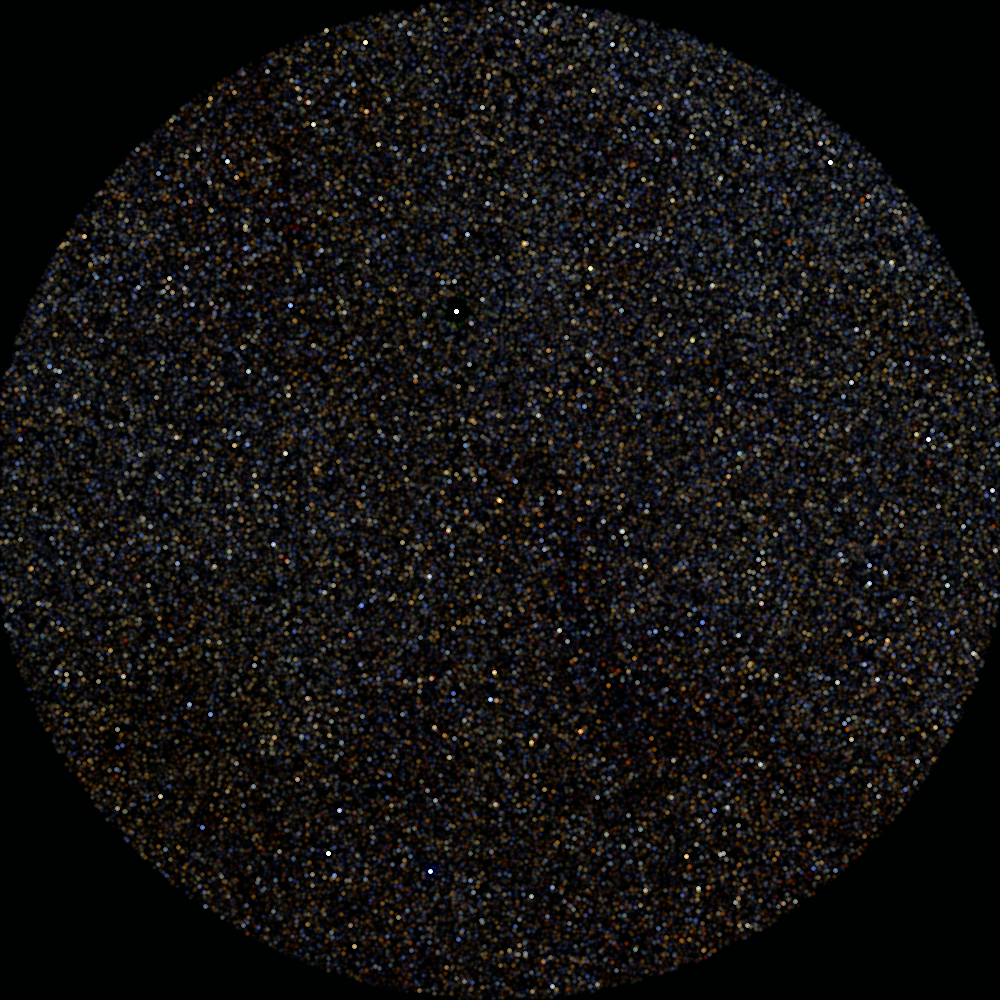# mCatMap

Description:
mCatMap is a point-source imaging program. The user defines a general output FITS image, and its pixels are populated from a table of point sources. The source fluxes (or just source counts) from the table are added into the appropriate pixel to create an output image.
Syntax:
mCatMap [-c column] [-m refmag] [-d level] [-w size] in.tbl out.fits hdr.template

Switches:

-c column
Name of the table column that contains flux levels. If not specified, pixels will be populated with source counts rather than summed flux values.
-m refmag
Set a reference magnitude to use when calculating fluxes.
-d level
Turn on debugging to the specified level (1-3)
-w size
Set a spread size for point sources (default is to use no spread). Allowed values are 3 or 5.

Arguments:

in.tbl
out.fits
Path of output FITS file.
hdr.template
ASCII header template defining output FITS file.
Results:
[struct stat="OK", time=seconds]

Examples:

To create an image showing the 75,000 2MASS point source fluxes (2mass_psc.tbl) in a 2 square degree area defined by 2mass.hdr:

mCatMap -c j_m -m 15 -w 5 2mass_pt.tbl 2mass_j.fits 2mass.hdr
[struct stat="OK", time=1]

Note: The header file contains the same information as a FITS header but is looser on syntax (the lines do not need to be padded out to 80 characters). Headers like this can be created by hand or using programs like mHdr (for a region centered on a coordinate) or mMakeHdr (for a region bounding a set of sources/images) or can be pulled from an FITS image file (mGetHdr).

For the example below, we build images for each of the three 2MASS bands and then create a color composite PNG from them:

```   #!/bin/sh

mCatMap -c j_m -m 15. -w 5 2mass_psc.tbl 2mass_j.fits 2mass.hdr
mCatMap -c h_m -m 15. -w 5 2mass_psc.tbl 2mass_h.fits 2mass.hdr
mCatMap -c k_m -m 15. -w 5 2mass_psc.tbl 2mass_k.fits 2mass.hdr

mViewer -t     2 \
-blue  2mass_j.fits -2s max gaussian-log \
-green 2mass_h.fits -2s max gaussian-log \
-red   2mass_k.fits -2s max gaussian-log \
-out   2mass_psc.png
```Return Codes:

• [struct stat="OK", time=seconds]
• [struct stat="ERROR", msg="Usage: mCatMap [-c column] [-m refmag] [-d level] [-w size] in.tbl out.fits hdr.template"]
• [struct stat="ERROR", msg="Can't open input table in.tbl"]
• [struct stat="ERROR", msg="Can't find column 'ra'"]
• [struct stat="ERROR", msg="Can't find column 'dec'"]
• [struct stat="ERROR", msg="Can't find column 'column'"]
• [struct stat="ERROR", msg="Output wcsinit() failed"]